# spacesRGB User Guide

#### 2019-12-10

RGB spaces are confusing because for each RGB space, up to three RGB vectors are involved:

• linear scene RGB that represents light from a scene; this is optical in nature
• non-linear signal RGB encoded from scene RGB; this is electrical in nature
• linear display RGB that represents light emitted from a display; this is optical in nature

Without qualification, the non-linear signal RGB is usually meant. This is the case for grDevices::rgb(), grDevices::hsv(), grDevices::convertColor(), etc.

There are no strong package dependencies, but these packages are suggested:

• spacesXYZ  - used in some of the ACES Color transfer functions
• rgl  - used to make wireframe plots of 3D transfer functions
• microbenchmark  - for its high-precision timer

Similar packages: Package colorspace  has similar functionality, and is much faster because it is written in C; but it only supports one RGB space - sRGB. Package farver  is also much faster because it is written in C++. But it only supports one RGB space, which is probably sRGB.

And speaking of speed, this package is not fast enough to transform millions of RGB pixels. But it is fast enough to transform a thousand RGB patches; the function plotPatchesRGB() is available for that purpose. For the complicated ACES Transforms, it is hoped that this R package can be a completely independent check on other implementations.

# 1 Desktop Graphics and Basic Broadcast Television

The 16 RGB working spaces for desktop graphics at Lindbloom , and basic broadcast television standards (such as BT.709 and BT.2020), are illustrated in this diagram: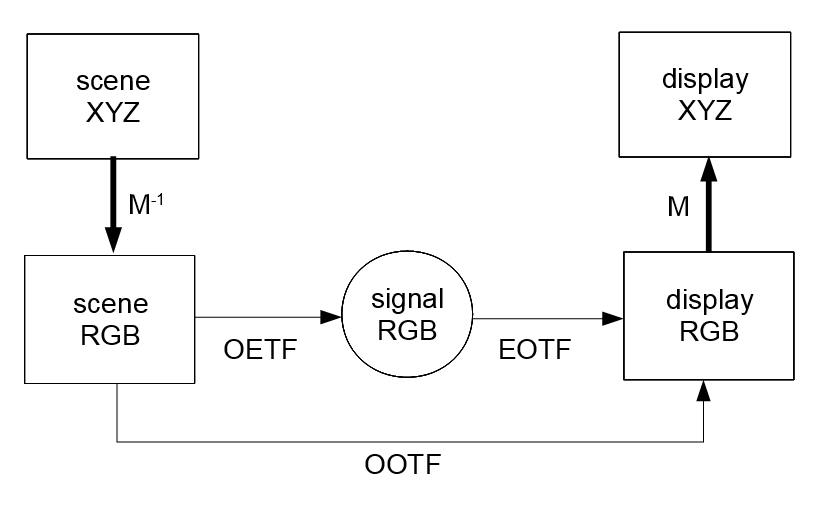Figure 1.1 - Desktop Graphics, and Broadcast Television

Scene XYZ is converted to scene RGB by a 3x3 matrix $$M^{-1}$$. The matrix is uniquely determined by the xy chromaticities of the RGB primaries and the XYZ of the whitepoint (3*2 + 3 = 9 total numbers); this is a fundamental fact from projective geometry, see  page 398. Display RGB is converted to display XYZ through multiplication by $$M$$. In this package $$M$$ and $$M^{-1}$$ are computed with full precision; the matrices that may be published in the standard are not used. All 4 vectors - scene RGB, scene XYZ, display RGB, and display XYZ - are called linear because they are all linear functions of the spectral energy distrubution of light.

The XYZ spaces are relative and normalized so that the maximum white has Y=1; there are no physical units for XYZ. Compare this with display XYZ in ACES Color.

The terminology below is mostly taken from BT.709 , BT.1886 , and BT.2100 .

In this package, both linear RGB vectors are normalized to the cube [0,1]$$^3$$; they are usually thought of as optical in nature. Signal RGB can be thought of as electrical in nature. It is common to think of this signal as an 8-bit number stored in the frame buffer, so the cube [0,255]$$^3$$ is very commmon for signal RGB. This package allows the user to specify any positive number as the upper limit of the interval. The relevant function argument is maxSignal.

In the above figure the thin arrows indicate that the transforms are univariate and operate independently on each channel and in the same way. These transforms - OETF, EOTF, and OOTF - are called transfer functions. The thick arrows indicate that the transform is multivariate; in this diagram they are the 3x3 matrix transforms.

The conversion function from optical scene RGB (linear) to electrical signal RGB (non-linear) is called the Opto-Electronic Transfer Function (OETF). Each primary component (R,G, and B) is transformed independently and has the same OETF. In this section, the OETF is always defined as a map of [0,1] to itself. The classical OETF is the “$$1/\gamma$$ power law”. For Adobe RGB, the OETF is classical with $$\gamma = 563/256 \approx 2.2$$. For the most popular RGB space - sRGB - the OETF is a continuous function defined in 2 pieces. In ICC profiles, the OETF is called a Tone Response Curve (TRC), or shaper curve.

All the transfer functions in this section are continuous and strictly increasing functions that take [0,1] to itself. All transfer functions in this section are either power laws, or well-approximated by power laws. The exponent giving the best match is called the effective gamma, the approximate gamma, or the best-fit exponent for the transfer function. The function summaryRGB() displays the best-fit exponent for the transfer functions of all these basic RGB spaces.

The conversion function from electrical signal RGB (non-linear) to optical display RGB (linear) is called the Electro-Optical Transfer Function (EOTF). Each primary component (R,G, and B) is transformed independently and has the same EOTF. The classical EOTF is the “$$\gamma$$ power law”. That is $$R' = R^\gamma$$, where $$R'$$ is display Red and $$R$$ is signal Red. In this section, the EOTF is always the inverse of the OETF; i.e. EOTF=OETF$$^{-1}$$.

The Opto-optical Transfer Function (OOTF) is the OETF followed by the EOTF. This is called their composition and in this User Guide we will write: $$\text{OOTF} = \text{OETF} \otimes \text{EOTF}$$, following BT.2100 . In the R code we use the symbols * or %X%. Since EOTF=OETF$$^{-1}$$, the OOTF is the identity, i.e. trivial. In some contexts one says that the system gamma is 1, or that the end-to-end exponent is 1. This implies that scene RGB and display RGB are the same, and that scene XYZ and display XYZ are the same. The OOTF is also called the system transfer function.

theSpace = getRGB('sRGB')
par( omi=c(0,0,0,0), mai=c(0.6,0.55,0.1,0.1) )
plot( theSpace$OETF, main='', ylab='', color='red' ) plot( theSpace$EOTF, add=TRUE, color='blue' ) ; plot( theSpace$OOTF, add=TRUE, color='black' ) legend( 'topleft', legend=c('OETF','EOTF','OOTF'), bty='n', lwd=2, col=c('red','blue','black') )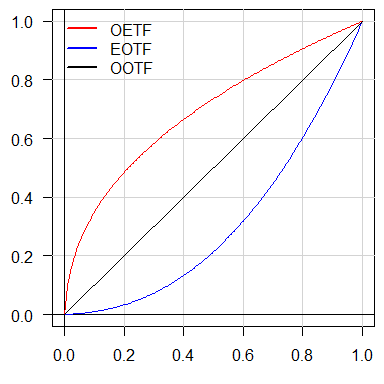Figure 1.2 The 3 Transfer Functions of sRGB To summarize, the RGB spaces in this section all have these simplifying properties: 1. The scene primaries and whitepoint are the same as the display primaries and whitepoint. These are expressed in CIE xy (or possibly XYZ for the whitepoint). This is equivalent to the fact that the 2 3x3 matrices in Figure 1.1 are inverses of each other. 2. All transfer functions are univariate, with domain and range the interval [0,1], and operate in the same way on all 3 channels. 3. The OOTF is the identity, or equivalently, the scene RGB and display RGB vectors are equal. These 3 properties are enjoyed by all 16 working RGB spaces at Lindbloom . This package includes three of these working spaces: sRGB, Adobe RGB, and Apple RGB. The others can be easily added by the user using installRGB(), see Appendix A for how this works. The 3 properties are also enjoyed by these RGB spaces: ProPhoto RGB, BT.709, BT.2020, and 240M, which are also included. There are some close similarities between some of these spaces. BT.709 (created 1990) and sRGB (created 1996) have the same primaries and whitepoint, but different transfer functions. sRGB copied its primaries and whitepoint from BT.709. BT.709 and BT.2020 have very close transfer functions, differing only in precision. HD+2.4 has the same primaries and OETF as BT.709, but a different EOTF. Regarding property 3, it turns out that color reproduction for human vision is better when property 3 is not satisfied. In the next section we present an RGB space with a non-trivial OOTF, i.e. where the system gamma is not 1. # 2 Advanced Broadcast Television Due to the Hunt effect and the Stevens effect, color reproduction for human vision is better when the OOTF is not the identity. For an illustration see BT.2390  Figure 8, and for further discussion see  and . The recommendation in BT.1886  is to use the OETF from BT.709  and for the EOTF use a pure power law with $$\gamma=2.4$$ (with optional black offset). The resulting RGB space “HD+2.4” is installed like this: prim = matrix( c(0.64,0.33, 0.30,0.60, 0.15,0.06, 0.3127,0.3290), 4, 2, byrow=TRUE ) installRGB( 'HD+2.4', scene=prim, OETF=BT.709.EOTF^-1, EOTF=BT.1886.EOTF(), overwrite=TRUE )  And the resulting transfer functions look like this: theSpace = getRGB('HD+2.4') par( omi=c(0,0,0,0), mai=c(0.6,0.55,0.1,0.1) ) plot( theSpace$OETF, main='', ylab='', color='red' )
plot( theSpace$EOTF, add=TRUE, color='blue' ) ; plot( theSpace$OOTF, add=TRUE, color='black' )
legend( 'topleft', legend=c('OETF','EOTF','OOTF'), bty='n', lwd=2, col=c('red','blue','black') )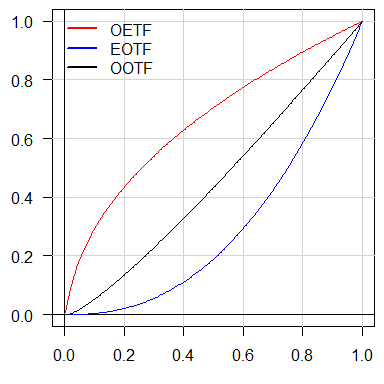Figure 2.1 The 3 Transfer Functions of HD+2.4

For the EOTF as given $$\gamma = 2.4$$ exactly. For the OETF $$\gamma \approx 1/1.96$$, and for the OOTF $$\gamma \approx 1.21$$ (both are approximate).

To summarize, this advanced RGB space has a non-trivial OOTF, but it still has these 2 simplifying properties:
1. The scene primaries and whitepoint are the same as the display primaries and whitepoint. These are expressed in CIE xy (or possibly XYZ for the whitepoint).
2. All transfer functions are univariate, with domain and range the interval [0,1], and operate in the same way on all 3 channels.

In section 4 we discuss ACES Color, which satisfies neither of these. But first, it is useful to discuss the transfer function implementation in more detail.

# 3 The TransferFunction S3 Class

Like most modern languages, R treats functions as “first-class citizens”. This allows us to “package” a function and its inverse function in a single object (a list) together with the domain and range of the function. The function must be injective, though this is only checked if the dimension is 1. What we have just described is a so-called elementary transfer function which is not exported and not accessible to the user. The inverse function is actually optional, and if the dimension is 1 an approximate inverse is constructed automatically (using stats::splinefun()). The dimension N is determined by the domain and range, which are 2xN matrices. Each matrix defines a (finite) box in R$$^N$$.

A TransferFunction is a list of elementary transfer functions. The constructor creates a list of length 1, and longer lists are created with composition() or equivalent infix operators: *, %X%, %O%, etc. In this User Guide we use only * and the symbol $$\otimes$$ (from BT.2100 ).

The package provides a number of built-in TransferFunction objects that are common in desktop publishing, broadcast television, and cinema. Some of these are parameterized, such as the function power.EOTF() that takes an argument for the power-law exponent $$\gamma$$. The functions composition() and inverse() allow building of more complex TransferFunctions from these simpler built-in ones.

Here is a simple example:

# create the squaring function on [0,1]
squaring  = TransferFunction( function(x) {x*x}, sqrt, domain=c(0,1), range=c(0,1) )
par( omi=c(0,0,0,0), mai=c(0.6,0.55,0.1,0.1) )
plot( squaring, main='', color='red' )
plot( inverse(squaring), add=TRUE, color='blue' )  # inverse is sqrt()
#   in the next line, power.EOTF(2) is also squaring, so comp should be identity
comp = squaring * inverse( power.EOTF(2.0) )
plot( comp, add=TRUE, color='black' )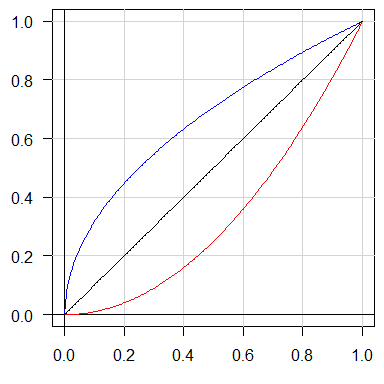Figure 3.1 Building TransferFunctions from Simpler Parts

transfer( comp, seq(0,1,length.out=11) )  # verify that comp is the identity
##   0.0 0.1 0.2 0.3 0.4 0.5 0.6 0.7 0.8 0.9 1.0

As in the above example, the transfer() method is used to actually transform numbers. Other useful methods are validate() to run a sequence of tests, print() to print basic properties (e.g. dimension, domain, and range) and also perform validation, and metadata() to store user-specific data with the object. There is inverse() which returns the inverse and equivalent postfix operator ^-1. See the man pages for more TransferFunction methods.

All the examples in this section are univariate, but multivariate functions are allowed. The rules for constructing univariate and multivariate TransferFunctions are a little different; again see the man pages. For multivariate examples, see the next section.

If one takes Figure 1.1 and removes the XYZ spaces, the remaining spaces form this commutative triangle: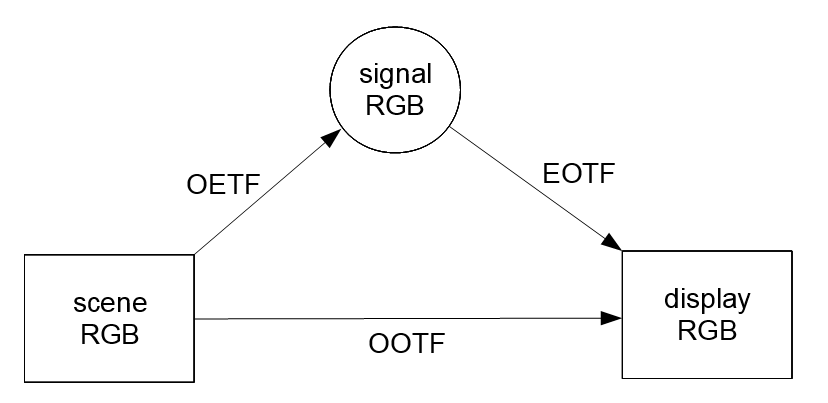Figure 3.2 - The Three Basic Transfer Functions

In the function installRGB(), the 3 transfer function arguments - OETF, EOTF, OOTF - can be ‘given’ or ‘not given’ (NULL). This yields 8 combinations, but only 6 are valid, as given in this table:

OETF EOTF OOTF installRGB() Action
given given given input is INVALID
given given - OOTF := OETF * EOTF
given - given EOTF := OETF^-1 * OOTF
- given given OETF := OOTF * EOTF^-1
given - - EOTF := OETF^-1 and OOTF := identity.TF
- given - OETF := EOTF^-1 and OOTF := identity.TF
- - given input is INVALID
- - - OETF = EOTF = OOTF := identity.TF

In the table NULL is replaced by -. If all 3 are given, it is INVALID because the triangle may not commute. If 2 functions are given, the 3rd is computed from those 2. If only 1 function is given, and it is EOTF or OETF, then it makes sense to make the other one the inverse of the given one, so that the OOTF is the identity. If only the OOTF is given, it is INVALID because there is no well-defined way to define the other two. If none are given, as in the last row, this might be useful for testing direct conversion between signal RGB and XYZ. The table contains a few cases where the inverse is used, but if the corresponding function is not invertible, installRGB() fails.

# 4 ACES Color - The Output Side

The Academy Color Encoding System is designed to seamlessly integrate digital video from any source, such as CGI, digital cinema cameras, and scanned celluloid film. See Academy Color Encoding System  and ACES Output Transform Details . All the R code for this section is based on that in aces-dev ; this is ACES v1.1 and subject to revision in the future.

The output side of ACES Color processing, is illustrated in this diagram: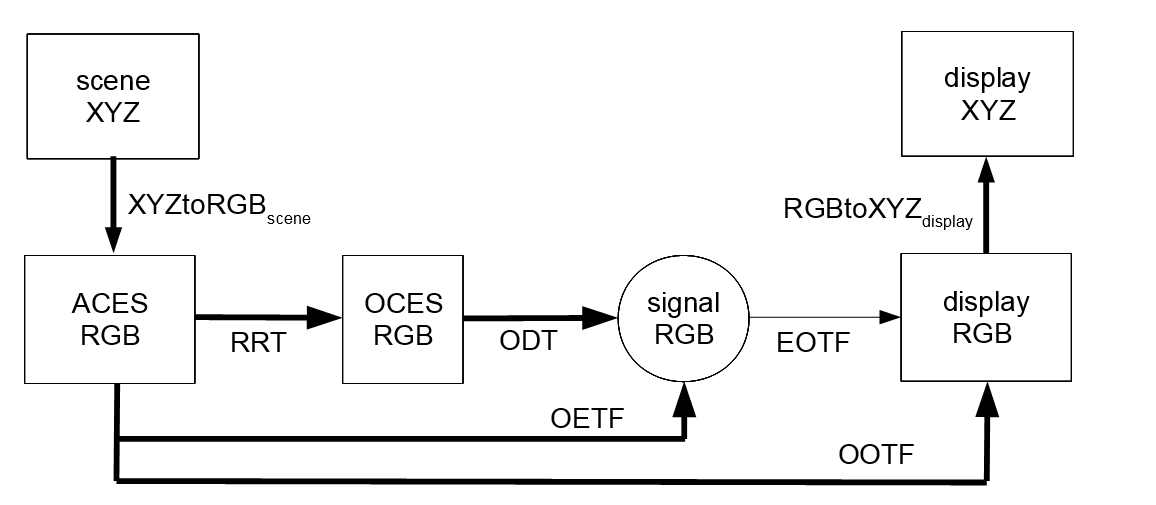Figure 4.1 - ACES Color Output (display)

ACES RGB (technically ACES2065-1 color space with AP0 primaries) is linear and scene-referred, with imaginary primaries, see . The complications of getting from camera signal to ACES RGB, often with a preferred camera “look”, is the input (capture) side of ACES (with IDT, LMT, CLF, …) and is currently outside the scope of this package. Unlike the RGB spaces in sections 1 and 2, these RGB values often exceed 1.0 for ordinary scenes. A perfect white diffuser in the scene is designed to be encoded with RGB=(1,1,1) and specular highlights can easily exceed this.

WARNING: What we call (non-linear) signal RGB is called Display RGB in ACES Output Transform Details . And what we call (linear) display RGB does not appear in .

In the above figure the thin arrow for EOTF indicates that the transform is univariate and operates independently on each channel and in the same way. Exception: the EOTF in the last transform in Appendix B - the hybrid log-gamma - is multivariate. For the other transforms, he thick arrows indicate that they are multivariate and 3D.

OCES RGB (Output Color Encoding Specification RGB) is display-referred but the display is not real - it is theoretical. This theoretical display has a dynamic range of 100 million to 1 and can reproduce any color. It uses the same imaginary AP0 primaries as ACES RGB.

The RRT is the Reference Rendering Transform from ACES to OCES; it is fixed and
“… the RRT is intended to account for the perceptual factors required to convert the scene referred ACES images to an output referred encoding associated with an very high dynamic range, extremely wide color gamut device in a dark surround.” - Alex Forsythe 

The ODT is the Output Device Transform for a real device. It includes the display primaries and whitepoint and other factors. Real world displays will still need calibration to exactly match the ideal color space of each ODT.

The ODT may be split further using the concept of a Partial Output Device Transform or PODT, which is unique to this package. The split is $$\text{ODT} = \text{PODT} \otimes \text{EOTF}^{-1}$$ for some standard $$\text{EOTF}$$. The function general.PODT() returns a PODT which is used for all of the ODT examples in Section 7.1. In these examples $$\text{OETF} = \text{RRT} \otimes \text{ODT} = \text{RRT} \otimes \text{PODT} \otimes \text{EOTF}^{-1}$$. This ODT split is not shown in the above figure.

In the examples in Section 7.2, the OOTF and EOTF are given and $$\text{OETF} := \text{OOTF} \otimes \text{EOTF}^{-1}$$. The function general.OOTF() is used for these examples, and there is no explicit RRT or ODT.

Since the display primaries and whitepoint are different than those of ACES RGB, the matrices $$\text{XYZtoRGB}_\text{scene}$$ and $$\text{RGBtoXYZ}_\text{display}$$ are not inverses of each other, as they are in Figure 1.1. Another difference with Figure 1.1 is that ACES display XYZ has physical units; the Y coordinate has units of $$cd/m^2$$ (aka $$nit$$).

Because of the complexity of ACES Color, calling a single instance of ACES an RGB “space” does not really do it justice. A more appropriate name might be an RGB “workflow” or “pipeline”.

## 4.1 Acknowledgements

I would like to thank Scott Dyer for supplying the high-precision data in the file test_values.txt in the folder tests, and Alex Forsythe for his encouragement to “dig into” ACES.

# 6 Appendix A - Built-in RGB Spaces

The package comes with a dictionary of 8 built-in RGB spaces; which are loaded from sysdata.rda. The code below shows how they are installed in that dictionary. Note that the argument scene can be a 4x2 matrix, or a list with a 6-vector and a 2-vector. Also note that the argument display is omitted, and the data is copied from scene. For details on this, see the man page for installRGB().

sRGB
installRGB( 'sRGB', scene=REC709_PRI, EOTF=sRGB.EOTF )


prim    = c(0.64,0.33,  0.21,0.71,  0.15,0.06)
white   = c( 0.3127, 0.3290 )      # D65


ProPhotoRGB
prim    = c(0.7347,0.2653,  0.1596,0.8404,  0.0366,0.0001)
white   = c( 0.3457,0.3585 )    # D50
installRGB( 'ProPhotoRGB', scene=list(prim,white), EOTF=ProPhotoRGB.EOTF )


AppleRGB
prim    = c(0.625,0.34,  0.28,0.595, 0.155,0.07)
white   = c( 0.3127, 0.3290 )
installRGB( 'AppleRGB', scene=list(prim,white), EOTF=1.8 )


BT.709
installRGB( 'BT.709', scene=REC709_PRI, EOTF=BT.709.EOTF )


BT.2020
installRGB( 'BT.2020', scene=REC2020_PRI, EOTF=BT.2020.EOTF )


240M
prim    = c(0.64,0.34,  0.31,0.595,  0.155,0.07 )
white   = c( 0.3127, 0.3290 )
installRGB( '240M', scene=list(prim,white), EOTF=SMPTE.240M.EOTF )


HD+2.4
installRGB( "HD+2.4", scene=REC709_PRI, OETF=BT.709.EOTF^-1, EOTF=2.4 )


# 7 Appendix B - Sample ODTs and Output Transforms for ACES

This Appendix has spacesRGB expressions for the output transforms available as presets to the user in ACES v1.1, see ACES Output Transform Details .

For examples of these transforms “in action” see the files test-ACES.R and test_values.txt in the folder tests.

## 7.1 Output Transforms with given OETF and EOTF

In  Sections 5.1 to 7.2 are 16 Output Transforms whose transfer functions are defined by their OETF and EOTF, and then $$\text{OOTF} := \text{OETF} \otimes \text{EOTF}$$. These tables present the OETF and EOTF using the S3 TransferFunction objects available in spacesRGB. The display primaries and whitepoints are easily extracted from the expressions for the OETF. Note that in the case of DCDM (Digital Cinema Distribution Master) in sections 5.7-9, which uses XYZ as its primaries, the argument display_pri=NULL.

Section 5.1 P3-D60
ACES Transform ID ODT.Academy.P3D60 48nits.a1.0.3
OETF RRT.TF * general.PODT( P3D60_PRI, Ymax=48 ) * power.OETF( 2.6 )
EOTF power.EOTF( 2.6 )
Section 5.2 P3-D65
ACES Transform ID ODT.Academy.P3D65 48nits.a1.1
OETF RRT.TF * general.PODT( P3D65_PRI, Ymax=48 ) * power.OETF( 2.6 )
EOTF power.EOTF( 2.6 )
Section 5.3 P3-D65 (D60 Simulation)
ACES Transform ID ODT.Academy.P3D65 D60sim 48nits.a1.1
OETF RRT.TF * general.PODT(P3D65_PRI,Ymax=48,observer=P3D60_PRI['W',]) * power.OETF(2.6)
EOTF power.EOTF( 2.6 )
Section 5.4 P3-D65 (Rec. 709 Limited)
ACES Transform ID ODT.Academy.P3D65 Rec709limited 48nits.a1.1
OETF RRT.TF * general.PODT(P3D65_PRI,Ymax=48,limiting_pri=REC709_PRI) * power.OETF(2.6)
EOTF power.EOTF( 2.6 )
Section 5.5 P3-DCI (D60 Simulation)
ACES Transform ID ODT.Academy.P3DCI D60sim 48nits.a1.1
OETF RRT.TF * general.PODT(P3DCI_PRI,Ymax=48,observer=P3D60_PRI['W',]) * power.OETF(2.6)
EOTF power.EOTF( 2.6 )
Section 5.6 P3-DCI (D65 Simulation)
ACES Transform ID ODT.Academy.P3DCI D65sim 48nits.a1.1
OETF RRT.TF * general.PODT(P3DCI_PRI,Ymax=48,observer=P3D65_PRI['W',]) * power.OETF(2.6)
EOTF power.EOTF( 2.6 )
Section 5.7 DCDM
ACES Transform ID ODT.Academy.DCDM.a1.0.3
OETF RRT.TF * general.PODT(NULL,Ymax=48) * DCDM.EOTF^-1
EOTF DCDM.EOTF
Section 5.8 DCDM (P3-D60 Limited)
ACES Transform ID ODT.Academy.DCDM P3D60limited.a1.1
OETF RRT.TF * general.PODT(NULL,Ymax=48,limiting_pri=P3D60_PRI) * DCDM.EOTF^-1
EOTF DCDM.EOTF
Section 5.9 DCDM (P3-D65 Limited)
ACES Transform ID ODT.Academy.DCDM P3D65limited.a1.1
OETF RRT.TF * general.PODT(NULL,Ymax=48,limiting_pri=P3D65_PRI) * DCDM.EOTF^-1
EOTF DCDM.EOTF
Section 6.1 Rec.709
ACES Transform ID ODT.Academy.Rec709 100nits dim.a1.0.3
OETF RRT.TF * general.PODT(REC709_PRI,Ymax=100,surround='dim') * BT.1886.EOTF()^-1
EOTF BT.1886.EOTF()
Section 6.2 Rec.709 (D60 Simulation)
ACES Transform ID ODT.Academy.Rec709 D60sim 100nits dim.a1.0.3
OETF RRT.TF * general.PODT(REC709_PRI,Ymax=100,observer=P3D60_PRI['W',],surround='dim') * BT.1886.EOTF()^-1
EOTF BT.1886.EOTF()
Section 6.3 Rec.2020
ACES Transform ID ODT.Academy.Rec2020 100nits dim.a1.0.3
OETF RRT.TF * general.PODT(REC2020_PRI,Ymax=100,surround='dim') * BT.1886.EOTF()^-1
EOTF BT.1886.EOTF()
Section 6.4 Rec.2020 (P3-D65 Limited)
ACES Transform ID ODT.Academy.Rec2020 P3D65limited 100nits dim.a1.1
OETF RRT.TF * general.PODT(REC2020_PRI,Ymax=100,limit=P3D65_PRI,surround='dim') * BT.1886.EOTF()^-1
EOTF BT.1886.EOTF()
Section 6.5 Rec.2020 (Rec.709 Limited)
ACES Transform ID ODT.Academy.Rec2020 P3D65limited 100nits dim.a1.1
OETF RRT.TF * general.PODT(REC2020_PRI,limit=REC709_PRI,surround='dim') * BT.1886.EOTF()^-1
EOTF BT.1886.EOTF()
Section 7.1 sRGB
ACES Transform ID ODT.Academy.RGBmonitor 100nits dim.a1.0.1
OETF RRT.TF * general.PODT(REC709_PRI,Ymax=100,surround='dim') * sRGB.EOTF^-1
EOTF sRGB.EOTF
Section 7.2 sRGB (D60 Simulation)
ACES Transform ID ODT.Academy.RGBmonitor D60sim 100nits dim.a1.0.1
OETF RRT.TF * general.PODT(REC709_PRI,Ymax=100,observer=P3D60_PRI['W',],surround='dim') * sRGB.EOTF^-1
EOTF sRGB.EOTF

None of these are pre-installed in the package. The resulting RGB space for last one - sRGB (D60 Simulation) - can be installed like this:

OETF = RRT.TF * general.PODT(REC709_PRI,observer=P3D60_PRI['W',],surround='dim') * sRGB.EOTF^-1
installRGB( 'sRGB_D60sim', scene=AP0_PRI, EOTF=sRGB.EOTF, OETF=OETF )

And the other 15 spaces can be installed in a similar way. Note that the display primaries REC709_PRI do not have to be passed to installRGB(), since they are automatically picked from the metadata of the OETF. See the man page for installRGB().

## 7.2 Output Transforms with given OOTF and EOTF

In  Sections 8.1 to 9.4 are 5 Output Transforms whose transfer functions are defined by their OOTF and EOTF, and then $$\text{OETF} := \text{OOTF} \otimes \text{EOTF}^{-1}$$. These tables present the OOTF and EOTF using the S3 TransferFunction objects available in spacesRGB. The display primaries and whitepoints are easily extracted from the expressions for the OOTF.

Section 8.1 P3-D65 ST2084 (108 nits)
ACES Transform ID RRTODT.Academy.P3D65 108nits 7.2nits ST2084.a1.1
OOTF general.OOTF( disp=P3D65_PRI, Ymid=7.2, Ymax=108 )
EOTF PQ.EOTF(10000/108)
Section 9.1 Rec.2020 ST2084 (1000 nits)
ACES Transform ID RRTODT.Academy.Rec2020 1000nits 15nits ST2084.a1.1
OOTF general.OOTF( disp=REC2020_PRI, Ymid=15, Ymax=1000 )
EOTF PQ.EOTF(10000/1000)
Section 9.2 Rec.2020 ST2084 (2000 nits)
ACES Transform ID RRTODT.Academy.Rec2020 2000nits 15nits ST2084.a1.1
OOTF general.OOTF( disp=REC2020_PRI, Ymid=15, Ymax=2000 )
EOTF PQ.EOTF(10000/2000)
Section 9.3 Rec.2020 ST2084 (4000 nits)
ACES Transform ID RRTODT.Academy.Rec2020 4000nits 15nits ST2084.a1.1
OOTF general.OOTF( disp=REC2020_PRI, Ymid=15, Ymax=4000 )
EOTF PQ.EOTF(10000/4000)
Section 9.4 Rec.2020 HLG (1000 nits)
ACES Transform ID RRTODT.Academy.Rec2020 1000nits 15nits HLG.a1.1
OOTF general.OOTF( disp=REC2020_PRI, Ymid=15, Ymax=1000 )
EOTF HLG.OETF()^-1 * HLG.OOTF(Lw=1000/1000)

In the final one, HLG.OETF() is univariate and HLG.OOTF(Lw=1) is multivariate with dimension 3. Thus the EOTF also has dimension 3. This is the only one of the 21 preset transforms that has a multivariate EOTF. The resulting RGB space can be installed like this:

installRGB( 'Rec.2020_HLG', scene=AP0_PRI, OOTF=general.OOTF(REC2020_PRI,Ymid=15,Ymax=1000),
EOTF=HLG.OETF()^-1 * HLG.OOTF(Lw=1) )

And the other 4 spaces can be installed in a similar way. Note that the display primaries REC2020_PRI do not have to be passed to installRGB(), since they are automatically picked from the metadata of the OOTF. See the man page for installRGB().

# 8 Session Information

R Under development (unstable) (2019-12-03 r77513)
Platform: i386-w64-mingw32/i386 (32-bit)
Running under: Windows 7 (build 7601) Service Pack 1

Matrix products: default

locale:
 LC_COLLATE=C                           LC_CTYPE=English_United States.1252
 LC_MONETARY=English_United States.1252 LC_NUMERIC=C
 LC_TIME=English_United States.1252

attached base packages:
 stats     graphics  grDevices utils     datasets  methods   base

other attached packages:
 spacesRGB_1.3-0

loaded via a namespace (and not attached):
 Rcpp_1.0.3           digest_0.6.22        spacesXYZ_1.0-4      magrittr_1.5
 evaluate_0.14        highr_0.8            rlang_0.4.1          stringi_1.4.3
 rmarkdown_1.18       tools_4.0.0          stringr_1.4.0        xfun_0.11
 yaml_2.2.0           compiler_4.0.0       microbenchmark_1.4-7 htmltools_0.4.0
 knitr_1.26